# Round, Round Up, or Round Down to Nearest 5 or .5 – Excel & Google Sheets

This tutorial will demonstrate how to round, round up, or round down to the nearest 5 or .5 in Excel and Google Sheets.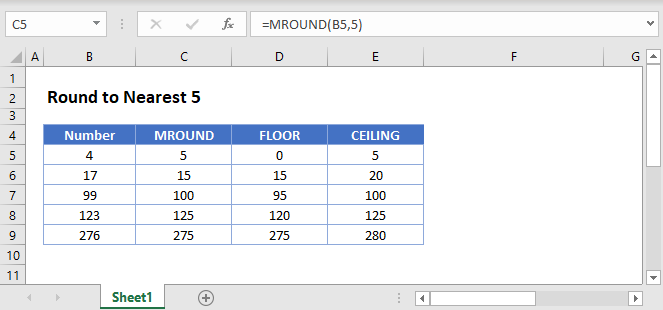## Round to Nearest 5

To round to the nearest 5, you can simply use the MROUND Function with multiple = 5.

``=MROUND(B3,5)``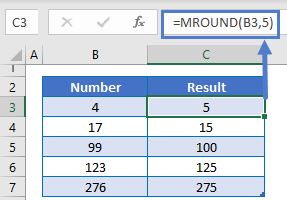By changing 5 to 50, you can round to the nearest 50 or you can use .5 to round to the nearest .5.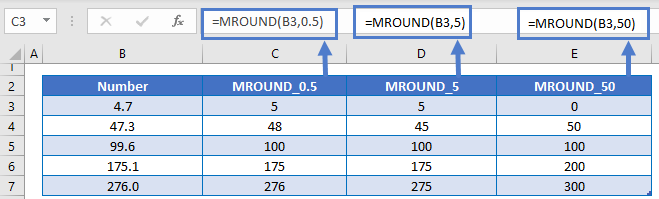## Round Up to Nearest 5

To round up to the nearest 5, use the CEILING Function with multiple = 5.

``=CEILING(B3,5)``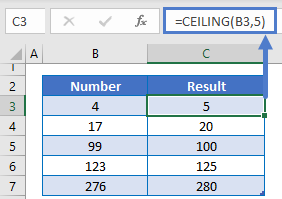Of course, you can use .5 to round up to the nearest .5 instead.

## Round Down to Nearest 5

To round down to the nearest 5, use the FLOOR Function with multiple = 5.

``=FLOOR(B3,5)``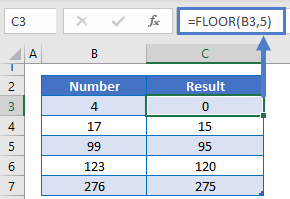## Round, Round Up, or Round Down to Nearest 5 or .5 in Google Sheets

All of the above examples work exactly the same in Google Sheets as in Excel.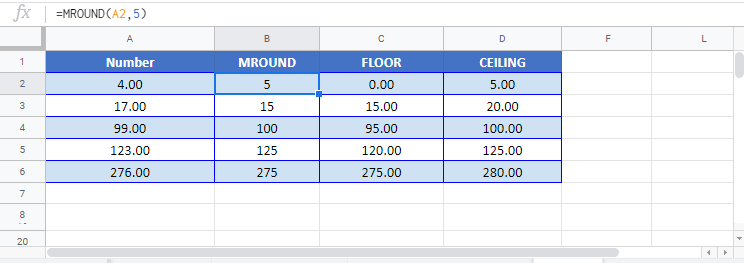### Excel Practice Worksheet

Practice Excel functions and formulas with our 100% free practice worksheets!